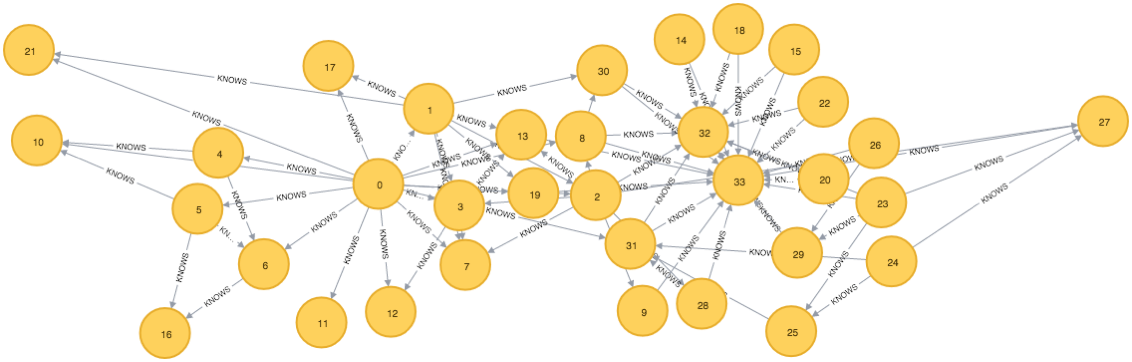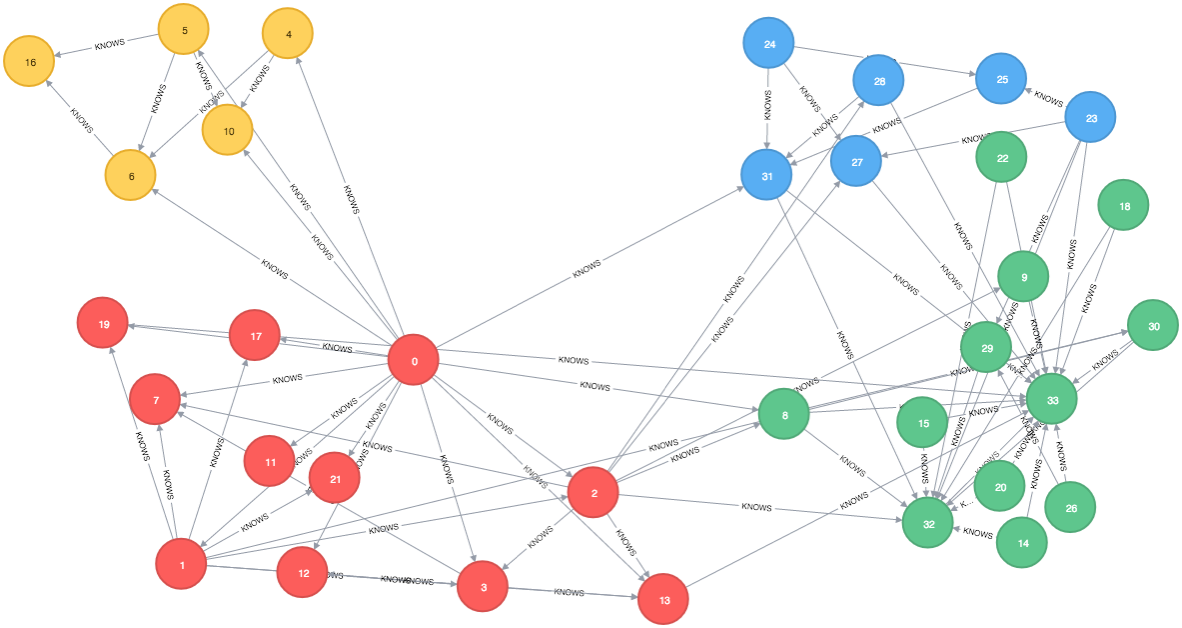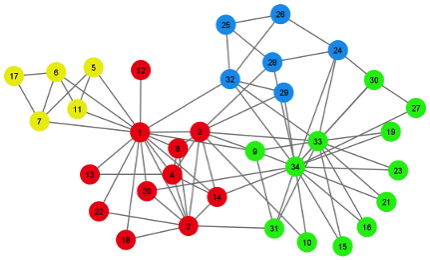# Neo4j: A procedure for the SLM clustering algorithm

In the middle of last year I blogged about the Smart Local Moving algorithmwhich is used for community detection in networks and with the upcoming introduction of procedures in Neo4j I thought it’d be fun to make that code accessible as one.

If you want to grab the code and follow along it’s sitting on the SLM repository on my github.

At the moment the procedure is hard coded to work with a KNOWS relationship between two nodes but that could easily be changed.

To check it’s working correctly I thought it’d make most sense to use the Karate Club data set described on the SLM home page. I think this data set is originally from Networks, Crowds and Markets.

I wrote the following LOAD CSV script to create the graph in Neo4j:

``````LOAD CSV FROM "file:///Users/markneedham/projects/slm/karate_club_network.txt" as row
FIELDTERMINATOR "\t"
MERGE (person1:Person {id: row})
MERGE (person2:Person {id: row})
MERGE (person1)-[:KNOWS]->(person2)``````Next we need to call the procedure which will add an appropriate label to each node depending which community it belongs to. This is what the procedure code looks like:

``````public class ClusterAllTheThings
{
@Context
public org.neo4j.graphdb.GraphDatabaseService db;

@Procedure
@PerformsWrites
public Stream<Cluster> knows() throws IOException
{
String query = "MATCH (person1:Person)-[r:KNOWS]->(person2:Person) \n" +
"RETURN person1.id AS p1, person2.id AS p2, toFloat(1) AS weight";

Result rows = db.execute( query );

ModularityOptimizer.ModularityFunction modularityFunction = ModularityOptimizer.ModularityFunction.Standard;
Network network = Network.create( modularityFunction, rows );

double resolution = 1.0;
int nRandomStarts = 1;
int nIterations = 10;
long randomSeed = 0;

double modularity;

Random random = new Random( randomSeed );

double resolution2 = modularityFunction.resolution( resolution, network );

Map<Integer, Node> cluster = new HashMap<>();
double maxModularity = Double.NEGATIVE_INFINITY;

for ( int randomStart = 0; randomStart < nRandomStarts; randomStart++ )
{
network.initSingletonClusters();

int iteration = 0;
do
{
network.runSmartLocalMovingAlgorithm( resolution2, random );
iteration++;

modularity = network.calcQualityFunction( resolution2 );
} while ( (iteration < nIterations) );

if ( modularity > maxModularity )
{
network.orderClustersByNNodes();
cluster = network.getNodes();
maxModularity = modularity;
}
}

for ( Map.Entry<Integer, Node> entry : cluster.entrySet() )
{
Map<String, Object> params = new HashMap<>();
params.put("userId", String.valueOf(entry.getKey()));

db.execute("MATCH (person:Person {id: {userId}})\n" +
"SET person:`" + (format( "Community-%d`", entry.getValue().getCluster() )),
params);
}

return cluster
.entrySet()
.stream()
.map( ( entry ) -> new Cluster( entry.getKey(), entry.getValue().getCluster() ) );
}

public static class Cluster
{
public long id;
public long clusterId;

public Cluster( int id, int clusterId )
{
this.id = id;
this.clusterId = clusterId;
}
}
}``````

I’ve hardcoded some parameters to use defaults which could be exposed through the procedure to allow more control if necessary. The https://github.com/mneedham/slm/blob/master/src/main/java/org/neo4j/slm/Network.java#L69 function assumes it is going to receive a stream of rows containing columns 'p1', 'p2' and 'weight' to represent the 'source', 'destination' and 'weight' of the relationship between them.

We call the procedure like this:

``CALL org.neo4j.slm.knows()``

It will return each of the nodes and the cluster it’s been assigned to and if we then visualise the network in the neo4j browser we’ll see this:``````\$ mvn clean package